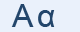Greek Letter Alpha (Άλφα - Α)A is the first letter of the Greek alphabet and it derives from the Egyptian hieroglyphic for a horned ox's head, by way of the semitic "aleph", which today doesn't look at all ox-like. Contemporary "A", like its Greek ancestor, has the horns pointed downward.

"A" Letter in mathematics and in science

α represents:

• the first angle in a triangle.
• one root of a quadratic equation, β represents the other
• the statistical significance of a result
• the false positive rate in statistics
• the fine structure constant in physics which characterizes the strength of the electromagnetic interaction
• Alpha particles that consist of two protons and two neutrons bound together into a particle identical to a helium nucleus; (He+2)
• Angular acceleration in physics
• the linear thermal expansion coefficient

Greek words with "α"

αλήθεια, γάτα